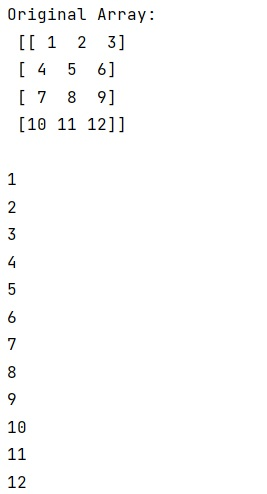# How to loop through 2D NumPy array using x and y coordinates without getting out of bounds error?

Given a 2D NumPy array, we have to loop through 2D NumPy array using x and y coordinates without getting out of bounds error.
Submitted by Pranit Sharma, on February 19, 2023

NumPy is an abbreviated form of Numerical Python. It is used for different types of scientific operations in python. Numpy is a vast library in python which is used for almost every kind of scientific or mathematical operation. It is itself an array which is a collection of various methods and functions for processing the arrays.

## Looping through 2D NumPy array using x and y coordinates

Suppose that we are given a 2D numpy array and we need to loop over this numpy array using the x and y coordinates. By x and y coordinates, we mean that we need to loop extract all the columns of first row and so on.

For this purpose, we will loop over the numpy array using numpy.nindex() where we will pass the shape of the array.

Let us understand with the help of an example,

## Python code to loop through 2D NumPy array using x and y coordinates without getting out of bounds error

```# Import numpy
import numpy as np

# Creating a numpy array
arr = np.array([[1,2,3],[4,5,6],[7,8,9],[10,11,12]])

# Display original array
print("Original Array:\n",arr,"\n")

# looping over the array
for iy, ix in np.ndindex(arr.shape):
print(arr[iy, ix])
```

Output: